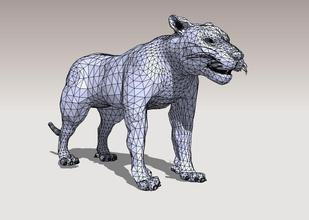``````attribute vec4 a_Position;
uniform vec4 u_Color;
void main(){
gl_Position = a_Position;
gl_PointSize = 10.0;
}
``````

``````precision highp float;
uniform vec4 u_Color;
void main(){
gl_FragColor = u_Color;
}
``````

`vertex shader` (顶点着色器). 控制点的位置和大小.

`fragment shader` (片元着色器).控制点的颜色.

### 关于canvas标签

``````var canvas =document.getElementById('canvas');
var ctx=canvas.getContext("2d");

...
...
ctx.lineTo()
``````

context2d api 是在canvas上绘图的最简单的 API. 我们通过调用 `canvas.getContext("2d")` 获得了一个 context2d 对象`ctx`,之后的绘图工作 只需要调用ctx下的相应绘图函数就行.

webgl 下图像也是显示在canvas中的,获取webgl对象的方式与获取context2d对象类似:

``````var canvas =document.getElementById('canvas');
var gl=canvas.getContext("webgl");
``````

``````var vertexShader = gl.createShader(gl.VERTEX_SHADER);

``````

``````var program = gl.createProgram();
gl.useProgram(program);``````

``````g++ -o a.cpp
g++ -o b.cpp``````

.o文件我们不能直接用,我们还需要把他们链接起来

``g++ a.o b.o -o test.exe``